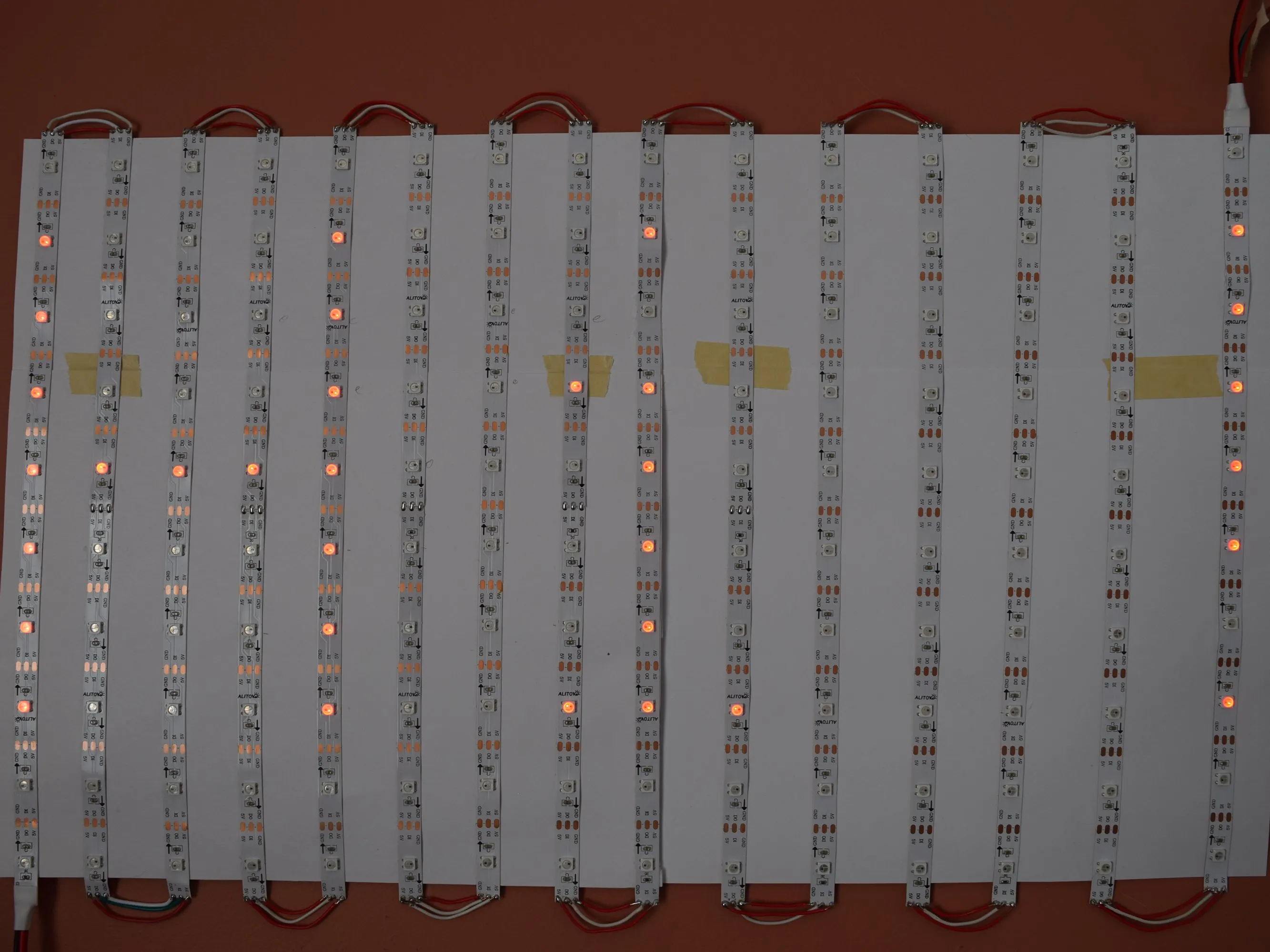Project tutorialHow to Make a NeoPixel Matrix © GPL3+

Build a simple NeoPixel matrix with these simple steps!

• 7,223 views
• 16 respects

Necessary tools and machinesSoldering iron (generic)

Apps and online services

The Matrix

No, not the movie, the NeoPixel matrix! To make a matrix, just buy one strip of individually addressable NeoPixel LEDs. Uncoil the strip, and take note of the arrows on it. You can only attach them in ONE DIRECTION, you could ruin them if the orientation is wrong.

I used a 30 LED/m 5m strip and cut them into strips of ten LEDs each.

I then started laying down the strips on a piece of cardstock, alternating orientations.

I then cut wires to the correct lengths and soldered them to the NeoPixel terminals.

After doing that 14 times, my matrix was finally done! Next, I checked with a multimeter to ensure that there were no short-circuits, as a short-circuit will fry the Nano, the matrix, and maybe your USB port (I know from experience). Upload the neomatrix test sketch with the "NEO_BOTTOM + NEO_LEFT + NEO_COLUMNS + NEO_ZIGZAG" parameters. If it scrolls "howdy" across the display, you're done!

Check out my other projects for more NeoPixel matrix ideas!

Code

Arduino CodeC/C++
Copy and paste
// Adafruit_NeoMatrix example for single NeoPixel Shield.
// Scrolls 'Howdy' across the matrix in a portrait (vertical) orientation.

#ifndef PSTR
#define PSTR // Make Arduino Due happy
#endif

#define PIN 6

// MATRIX DECLARATION:
// Parameter 1 = width of NeoPixel matrix
// Parameter 2 = height of matrix
// Parameter 3 = pin number (most are valid)
// Parameter 4 = matrix layout flags, add together as needed:
//   NEO_MATRIX_TOP, NEO_MATRIX_BOTTOM, NEO_MATRIX_LEFT, NEO_MATRIX_RIGHT:
//     Position of the FIRST LED in the matrix; pick two, e.g.
//     NEO_MATRIX_TOP + NEO_MATRIX_LEFT for the top-left corner.
//   NEO_MATRIX_ROWS, NEO_MATRIX_COLUMNS: LEDs are arranged in horizontal
//     rows or in vertical columns, respectively; pick one or the other.
//   NEO_MATRIX_PROGRESSIVE, NEO_MATRIX_ZIGZAG: all rows/columns proceed
//     in the same order, or alternate lines reverse direction; pick one.
//   See example below for these values in action.
// Parameter 5 = pixel type flags, add together as needed:
//   NEO_KHZ800  800 KHz bitstream (most NeoPixel products w/WS2812 LEDs)
//   NEO_KHZ400  400 KHz (classic 'v1' (not v2) FLORA pixels, WS2811 drivers)
//   NEO_GRB     Pixels are wired for GRB bitstream (most NeoPixel products)
//   NEO_RGB     Pixels are wired for RGB bitstream (v1 FLORA pixels, not v2)

// Example for NeoPixel Shield.  In this application we'd like to use it
// as a 5x8 tall matrix, with the USB port positioned at the top of the
// Arduino.  When held that way, the first pixel is at the top right, and
// lines are arranged in columns, progressive order.  The shield uses
// 800 KHz (v2) pixels that expect GRB color data.
NEO_MATRIX_BOTTOM     + NEO_MATRIX_LEFT +
NEO_MATRIX_COLUMNS + NEO_MATRIX_ZIGZAG,
NEO_GRB            + NEO_KHZ800);

const uint16_t colors[] = {
matrix.Color(255, 0, 0), matrix.Color(0, 255, 0), matrix.Color(0, 0, 255) };

void setup() {
matrix.begin();
matrix.setTextWrap(false);
matrix.setBrightness(40);
matrix.setTextColor(colors);
}

int x    = matrix.width();
int pass = 0;

void loop() {
matrix.fillScreen(0);
matrix.setCursor(x, 0);
matrix.print(F("Howdy"));
if(--x < -36) {
x = matrix.width();
if(++pass >= 3) pass = 0;
matrix.setTextColor(colors[pass]);
}
matrix.show();
delay(100);
}

Schematics8x8 Dot Matrix

Project tutorial by robocircuits

• 1,062 views
• 4 respects

48 x 8 Scrolling LED Matrix using Arduino.

Project tutorial by Prasanth K S

• 18,921 views
• 24 respects

NeoPixel Lightsabers w/ Party Modes -- Arduino-Controlled

Project tutorial by Modustrial Maker

• 8,427 views
• 35 respects

NeoMatrix Arduino Pong

Project tutorial by Arduino “having11” Guy

• 4,649 views
• 19 respects

Controlling an LED Matrix with Arduino Uno

Project tutorial by Igor Fonseca Albuquerque

• 32,892 views